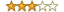# Statistics-Regression reviews

### Statistics-Regression (0.53)I have used Statistics::Regression in a couple of projects. I find it useful, but flawed.

* provides most of the basic multivariate linear regression functionality, including getting standard errors for the fitted parameters
* does NOT hardwire a constant
* nice Perl API to add one point at a time (I haven't tried the provide-all-data-at-once API)
* provides linearcombination_variance()
* uses Gentleman's algorithm, which seems to be numerically quite robust

* documentation is a bit skimpy, e.g., it never explicitly says that the usage for include() is include(y, [x0,x1,x2,...,xk]), rather I had to reverse-engineer this from the examples
* does not seem to provide any way to get the covariance matrix of the fitted parameters
* no documentation at all for linearcombination_variance() :(
* doesn't offer any help in diagnosing problems caused by a linearly-dependent set of basis fns (this would require an SVD-based fit)
* There must be at least 2 independent variables -- attempting to do a regression with only a single independent variable [i.e., attempting to estimate the parameter c given a set of (x,y) data points and the model y = c*x ] yields a run-time error message that this isn't allowed. This restriction is unfortunate (the mathematical formalism is ok with 1 independent variable, and some applications generate N-variable fits where N isn't known in advance). What's worse, this restriction is undocumented.

I have been using Statistics::Regression for about a year in an ongoing project (which totals around 2500 lines of Perl), and generally been fairly happy with it. (Not having a covariance matrix has been a bit awkward, but I could live with(out) it.)

I haven't tried the fancy "pretty-print the regression" routines.

Alas, I've just hit the "must have at least 2 independent variables" restriction, so it looks like I'll have to convert my code to use a different regression package (maybe Math::GSL::Multifit).

### Statistics-Regression (0.15)Title: good job, but not yet done

1. Overall Impression: This package is useful and has fewer bugs than might be expected in a 0.x version.

2. The sigmasq method does not work. Here's the error message that I got when I tried to use it:

Internal Error: yelement is undef at C:/Perl/site/lib/Statistics/Regression.pm line 265.

3. For the sake of efficiency, there should be a mechanism for adding multiple observations at a single shot.

4. The documentation should specify the formulas that are being used or at least provide references.

5. It would be nice to have a method that returns the coefficients, R^2, and sigma^2 all at a single shot (returning a reference to the coefficient vector).